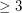# Reconstruction conjecture

 Importance: Outstanding ✭✭✭✭
 Author(s): Kelly, Paul J. Ulam, Stanislaw M.
 Subject: Graph Theory
 Keywords: reconstruction
 Posted by: zitterbewegung on: October 18th, 2007

The deck of a graphis the multiset consisting of all unlabelled subgraphs obtained fromby deleting a vertex in all possible ways (counted according to multiplicity).

Conjecture   If two graphs onvertices have the same deck, then they are isomorphic.

See Wikipedia's Reconstruction Conjecture for more on this problem.

## Bibliography

*[K] P. J. Kelly, A congruence theorem for trees, Pacific J. Math., 7 (1957), 961–968.

*[U] S. M. Ulam, A collection of mathematical problems, Wiley, New York, 1960.

* indicates original appearance(s) of problem.

### A strategy for simple graphs?

How about 1) Prove for a 4-node, or tetrahedral graph. 2) Demonstrate that all graphs with > 4 nodes are composed of multiple overlapping tetrahedrons 3) Figure out how coupled tetrahedra function when nodes are deleted. 4) Induct on number of tetrahedra in graph. ?

Obviously (3) is the tough part, but why should it be impossible?

### correction and partial results

this should be on 3 or more vertices. False for digraphs, hypergraphs, and infinite graphs. It is open for simple graphs and multigraphs

### Question.

Does G have to be simple, or can it be a multigraph?